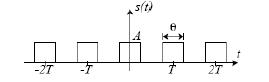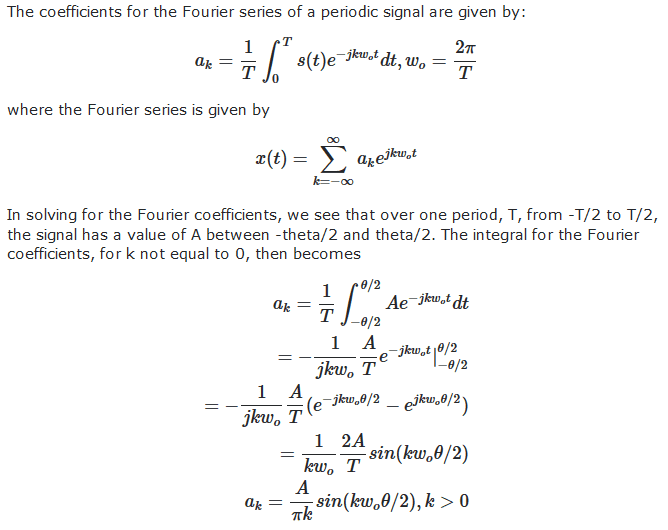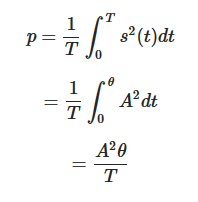# Evaluate Fourier series coefficients and power of a signal

• gruba

## Homework Statement

Derive the expression for coefficients of Fourier series in exponential form for the sequence of rectangular pulses (with amplitude A, period T and duration θ) shown in this image:Derive the expression for signal power depending on the coefficients of Fourier series.

-Fourier series

## The Attempt at a SolutionI don't know how to evaluate the signal power depending on the coefficients of Fourier series.

Average power is given by:Average power is not dependent on Fourier series coefficients. Is this a mistake in evaluation?
Is the signal power depending on the coefficients of Fourier series equal to the average power?

How to evaluate the signal power depending on the coefficients of Fourier series?

A few minor points first: in the equation defining the Fourier series, x(t) should be s(t); and θ = ωot , so you should have sin(/2) etc.
What happens when k = 0? And in your equation defining ak, why do you restrict k to be positive?

Average power is not dependent on Fourier series coefficients. Is this a mistake in evaluation?

Reference https://www.physicsforums.com/threa...es-coefficients-and-power-of-a-signal.907514/

I don't have full answers to your questions, but you may be expected to derive a power series whose sum is A2θ/T.

It should be obvious from symmetry that your Fourier series will be a sum of cosines; you could show this by combining the +k and -k terms. When you square it, you'll get terms proportional to cos(ot).cos(ot), where k and l are integers. Most of these terms will vanish when you integrate over one period, T. Which ones will remain, and what will they look like after integration? Without working it out, I think from that point everything might reduce to a sensible answer.

[...] and θ = ωot , so you should have sin(/2) etc. [...]

According to the problem statement, θ represents the fixed pulse duration. It is not a function of time $t$, rather it is a constant. Why the variable θ was chosen to represent the pulse duration is anybody's guess. But in this case I do not think the claim that θ = ωot is correct.

Power is directly obtainable from the Fourier coefficients. Hint: power in a given harmonic is independent of power in any of the other harmonics.
Cf. also Parseval's theorem.